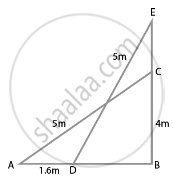# A 5 m long ladder is placed leaning towards a vertical wall such that it reaches the wall at a point 4 m high. If the foot of the ladder is moved 1.6 m towards the wall, then find the distance - Mathematics

Sum

A 5 m long ladder is placed leaning towards a vertical wall such that it reaches the wall at a point 4 m high. If the foot of the ladder is moved 1.6 m towards the wall, then find the distance by which the top of the ladder would slide upwards on the wall.

#### Solution

Let the length of the ladder = AC = 5 m

Let the height of the wall on which ladder is placed = BC = 4m.From right-angled ∆EBD,

Using the Pythagoras Theorem,

ED2 = EB2 + BD2

(5)2 = (EB)2 + (14)2  ......[BD = 1.4]

25 = (EB)2 + 1.96

(EB)2 = 25 – 1.96 = 23.04

EB = sqrt(23.04) = 4.8

Now, we have,

EC = EB – BC

= 4.8 – 4

= 0.8

Hence, the top of the ladder would slide upwards on the wall by a distance of 0.8 m.

Concept: Application of Pythagoras Theorem in Acute Angle and Obtuse Angle
Is there an error in this question or solution?

#### APPEARS IN

NCERT Mathematics Exemplar Class 10
Chapter 6 Triangles
Exercise 6.4 | Q 5 | Page 73

Share